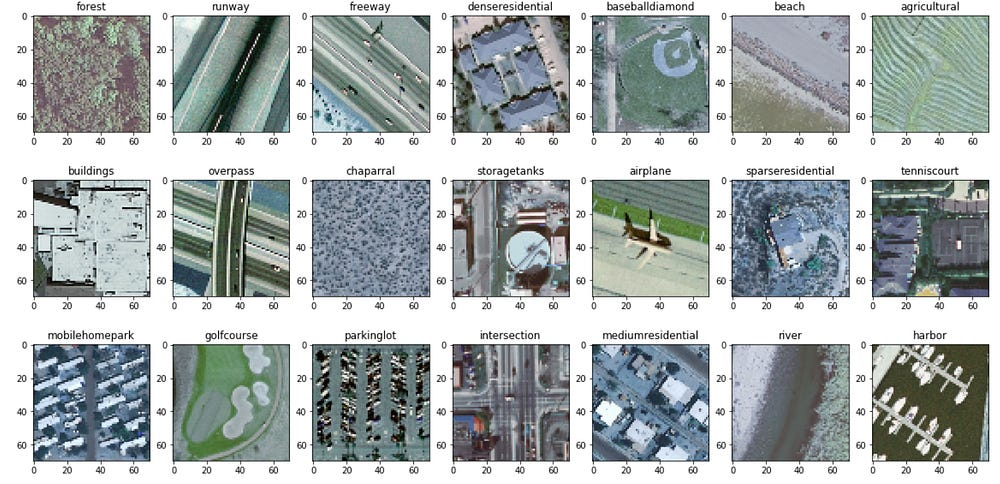# Land Use Classification using Convolutional Neural Networks

Source: Deep Learning on MediumLand Use Type From UCMerced Dataset

UCMerced Land Use Image Dataset consists of 21 land use class like Forest, Runaway, Freeway, Harbour and others. In this tutorials, we would like to share how to create Simple Convolutional Neural Networks program to classify Land Use. Before continue please kindly prepare software as follow:
Anaconda
– Keras Python Library

After collecting some libraries, lets begin to create the code with 6 step-by-step as follow:

1. Import Library requirement like Numpy, OpenCV, Matplotlib
`import numpy as npimport cv2import osimport reimport globimport matplotlib.pyplot as pltimport keras`
`from keras.models import Sequentialfrom keras.layers import Dense, Dropout, Activation, Flattenfrom keras.layers import Conv2D, MaxPooling2Dfrom keras.utils import np_utilsfrom keras import backend as Kfrom keras.optimizers import SGD`
`from sklearn.model_selection import train_test_splitfrom sklearn.metrics import classification_report`
`from keras.models import load_model`

2. Read Land Use Images

`path = os.path.abspath('[YOUR_FILE_NAME]')path = re.sub('[a-zA-Z\s._]+\$', '', path)dirs = os.listdir(path+'[YOUR_LAND_USE_PATH]')label = 0im_arr = []lb_arr = []X = []y = []for i in dirs: count = 0 for pic in glob.glob(path+'[YOUR_LAND_USE_PATH]'+i+'/*.tif'): im = cv2.imread(pic) im = cv2.resize(im,(70,70)) im = np.array(im) count = count + 1 X.append(im) y.append(label) if(count == 3): im_arr.append({str(i):im}) print("size "+str(i)+" : "+str(count)) label = label + 1 lb_arr.append(i)X = np.array(X)y = np.array(y);`

3. Image Pre-Processing

`X_train, X_test, y_train, y_test = train_test_split(X, y, test_size=0.33, random_state=42)`
`X_train = X_train.astype('float32') #set x_train data type as float32X_test = X_test.astype('float32') #set x_test data type as float32X_train /= 255 #change x_train value between 0 - 1X_test /= 255 #change x_test value between 0 - 1y_train = keras.utils.to_categorical(y_train, 21) #change label to binary / categorical: [1 0 0 0] = 0, [0 1 0 0] = 1, so ony_test = keras.utils.to_categorical(y_test, 21) #change label to binary / categorical`

4. Training Data

`model = Sequential() #model = sequential model.add(Conv2D(32, kernel_size=(3, 3),activation='relu',input_shape=(70,70,3)))model.add(MaxPooling2D(pool_size=(2,2)))model.add(Conv2D(32, (3, 3), activation='relu'))model.add(MaxPooling2D(pool_size=(2,2)))model.add(Dropout(0.25))model.add(Flatten()) #make layer flattenmodel.add(Dense(128, activation='relu'))model.add(Dropout(0.5))model.add(Dense(21, activation='softmax'))epochs = 25lrate = 0.01decay = lrate/epochssgd = SGD(lr=lrate, momentum=0.9, decay=decay, nesterov=False)model.compile(loss='categorical_crossentropy', optimizer=sgd, metrics=['accuracy'])print(model.summary())model.fit(X_train, y_train, validation_data=(X_test, y_test), epochs=epochs, batch_size=32)scores = model.evaluate(X_test, y_test, verbose=0)print("Accuracy: %.2f%%" % (scores*100))`

After Training 10 Epoch, accuracy of data reach 55.8% that good enough for classify 21 class.